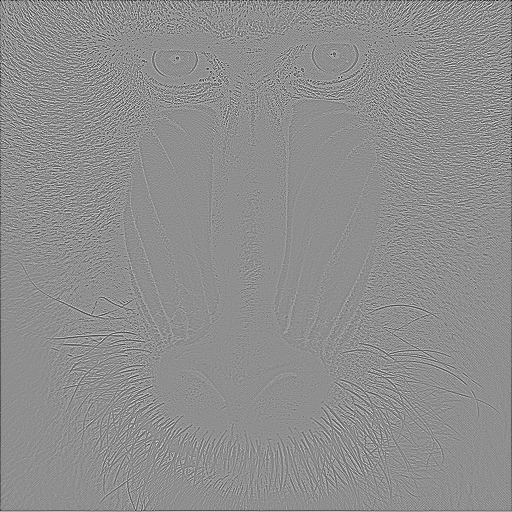Novelty Palliates Malaise

# npm

## ndarray-convolve

2.0.0 • Public • Published

# ndarray-convolve

## Example

This produces the following array:## Install

``````npm install ndarray-convolve
``````

## API

### `convolve( ... )`

Performs a convolution between two images with zero boundary conditions. As long as it does not cause unnecessary cropping, the kernel (`b`) will be assumed to have its origin in the center of the kernel (for even kernels, slightly to the right of the center, for example `[1,2,3,4]` would be assumed to have it origin at 3). There are four ways you can call this function:

#### `convolve(a, b)`

Convolves `a` and `b` storing the result in `a`

#### `convolve(out, a, b)`

Convolves `a` and `b` storing the result in `out`

#### `convolve(a_r, a_i, b_r, b_i)`

Convolves two complex arrays storing the result in `a_r, a_i`

#### `convolve(out_r, out_i, a_r, a_i, b_r, b_i)`

Convolves two complex array storing the result in `out_r, out_i`

### `convolve.wrap( ... )`

Convolves two arrays with periodic boundary conditions. Same convention as `convolve`

### `convolve.correlate( ... )`

Cross correlates two arrays with zero boundary conditions. Same convention.

### `convolve.correlate.wrap( ... )`

Cross correlates two arrays with wrapped boundary conditions. Same convention again.

## Credits

(c) 2013 Mikola Lysenko. MIT License